# Python Program to Check if a Number is Positive, Negative or 0

In this tutorial you will learn about the Python Program to Check if a Number is Positive, Negative or 0 and its application with practical example.

In this tutorial, we will learn to create a Python Program to Check if a Number is Positive, Negative or Zero(0) using python Programming.

## Prerequisites

Before starting with this tutorial we assume that you are best aware of the following Python programming topics:

• Python Operator.
• Basic Input and Output
• Basic Python  programming.
• Python if-else statements.
• Python Loop.

## What is Positive Numbers ?

A number is called to be a positive number if it has a value Bigger than 0 (number >0), for example ‘1,2,3,4,5,6,7,8,9,10,11,12,13,..’,etc. All are Positive number and also natural numbers.

## What is Negative Numbers ?

A number is called to be a Negative number if it has a value smaller than 0 (number < 0), for example ‘-1,-2,-3,-4,-5,-6,-7,-8,-9,-10,-11,-12,-13,..’etc .All are Negative number and also natural  numbers.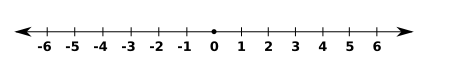## Python Program to Check if a Number is Positive, Negative or 0

In our program below we will check given number is Positive ,Negative or Zero number .First we would declared and initialized the required variables. Next, we would prompt user to input a number .Later we will find entered value is positive ,negative or Zero.

## Output

Inputted Number is Positive NumberNegative Number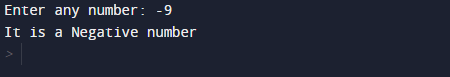Inputted Number is  Zero.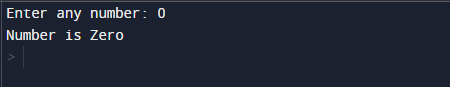In our program, we have first declared and initialized a set variables required in the program.

• number =taking year value from user.

First of all  we declare variable and take a value from user to check whether a entered number is  positive ,negative or Zero.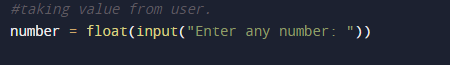After taking values from user we will check that if a number is greater than zero (number>0)then the given number is Positive .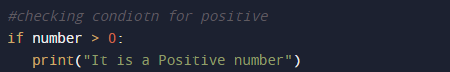Now  we will check that if a number is smaller than zero (number<0)then the given number is Negative .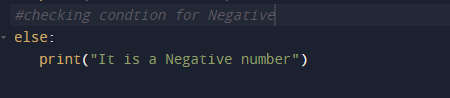Here, we going to use if…elif…else statement.Now at last if the given number is equal to Zero than the given number is zero.In this tutorial we have learn about the Python Program to Check if a Number is Positive, Negative or 0 and its application with practical example. I hope you will like this tutorial.